Weicheng Zhu

2013.12.06

# Fortran Compiler and Usage

## MinGW Installation

A contraction of "Minimalist GNU for Windows", is a minimalist development environment for native Microsoft Windows applications.

• MinGW: http://www.mingw.org/

• Provides a complete Open Source programming tool set which is suitable for the development of native MS-Windows applications, and which do not depend on any 3rd-party C-Runtime DLLs.
• A port of the GNU Compiler Collection (GCC), including C, C++, ADA and Fortran compilers;
• ...
• Install: check to install Fortran GNU Compiler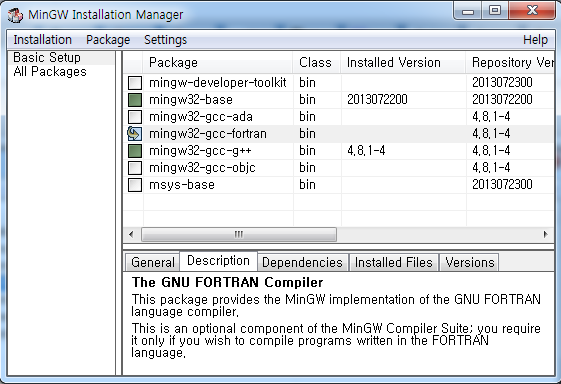• Set System PATH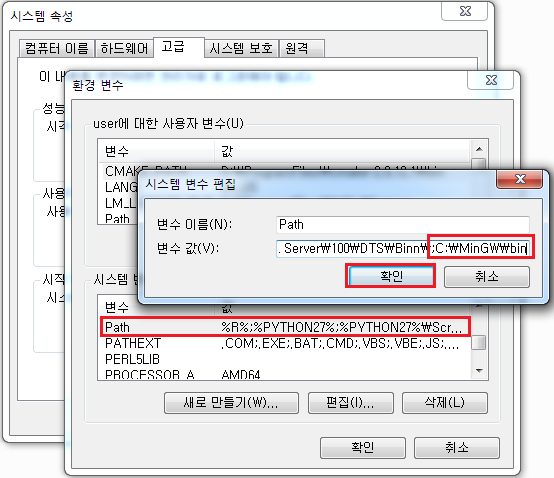• Confirm: in windows CMD command line, where is the equivalent of which in Linux. As shown in the following figure, there are two gfortran executable file in my system path, the first one will be used by default.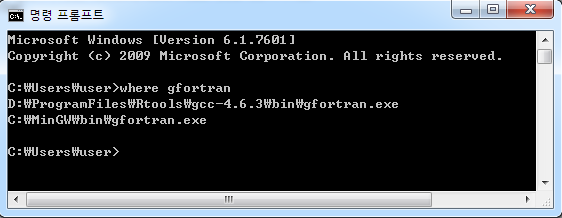## gfortran Usage

## The simplest way, default output is a.exe
gfortran tri-area.f90
## -o: specify the output name
gfortran tri-area.f90 -o TriArea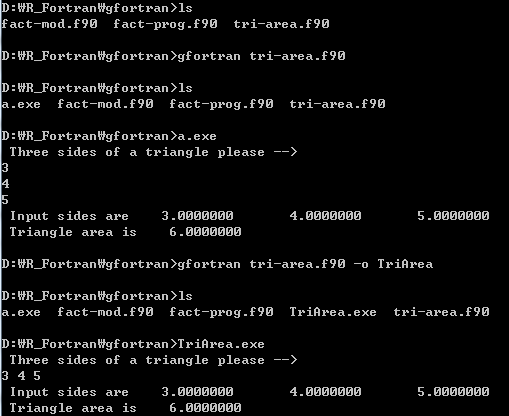• Example2
## 1. Straight forward
gfortran fact-prog.f90 fact-mod.f90 -o fact
## 2. Step by step
gfortran -c fact-mod.f90
gfortran -c fact-prog.f90
gfortran fact-prog.o fact-prog.o -o fact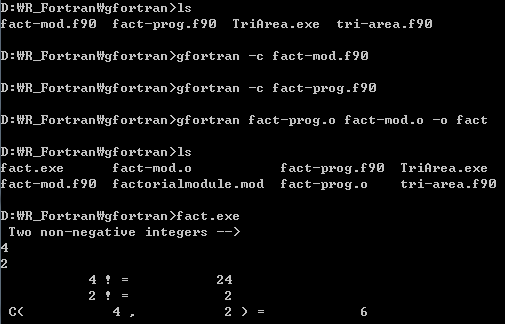Note1: In the step by step method, a factorialmodule.mod file is generated together with the fact-mod.o file. The former file is associated with the latter one, so if you delete the mod file, the last step will fail.

Note2: If you want to be an advanced developer, you should learn how to use command line and avoid using IDE if possible.

Note3: There are also some other popular Fortran compilers in Windows, such as Absoft Pro Fortran and Intel Fortran Compiler, which are all proprietary software. Since R uses gcc to build package and R itself in Windows, we only introduce gfortran compiler here.

# BLAS & LAPACK & ATLAS

## BLAS (Basic Linear Algebra Subprograms)

The Basic Linear Algebra Subprograms (BLAS) define a set of fundamental operations on vectors and matrices which can be used to create optimized higher-level linear algebra functionality.

Note: In this manual, we use the 'Prebuilt libraries'(check the 'Prebuilt dynamic libraries using Mingw' section described in Installation for Windows' above). Download the 32-bit dll files for both BLAS and LAPACK: libblas.dll and liblapack.dll. Of course, you can also build BLAS and LAPACK manually by yourself.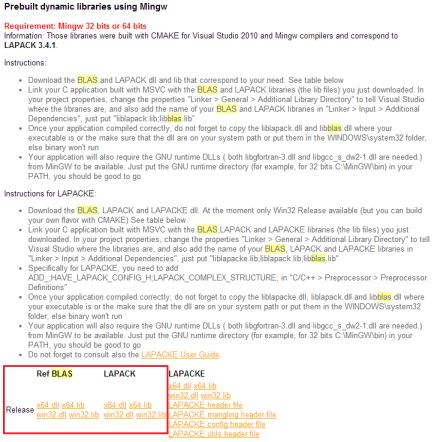• Examples: vector-vector calculation $$x^Tx$$; matrix-matrix calculation $$A^{-1}B$$
• Source location: gfortran/BlasStandalone/vector_dot.f and gfortran/BlasStandalone/matrix_sm.f
• Usage: Copy the downloaded libblas.dll to the BlasStandalone folder. Then, in the CMD command line, input make and enter, two executable files matrix_sm.exe and vector_dot.exe will be generated. Or you can input directly in the command line gfortran -o xxx.exe xxx.f -L. -lblas, where the -L. -lblas option means linking the libblas.dll file in the current directory to the program.
• Reference: sdot: http://www.netlib.org/blas/sdot.f; dtrsm: http://www.netlib.org/blas/dtrsm.f

Note: The gfortran compiler installed by Rtools lacks of some component for compiling standalone fortran program with BLAS and LAPACK, so you need to install MinGW first.

c vector_dot.f
c Example. Using BLAS Level 1 Routine
c vector-vector dot product
c Compute (1, 3, 5, 7, 9) * (10, 9, 8, 7, 6)
program dot_main
real x(10), y(10), sdot, res
integer n, incx, incy, i
n=5
incx=2
incy=1
do i = 1, 10
x(i) = i
y(i) = 11-i
end do
res = sdot(n, x, incx, y, incy)
print*,'SDOT =', res
end

c matrix_sm.f
c Example. Using BLAS Level 3 Routine
c matrix-matrix solve, solve(A)%*%B, where A is mxm, B is mxn
c         [1, 2, 3]      [1, 4]
c    A =  [2, 4, 5], B = [2, 5]
c         [3, 5, 6]      [3, 6]
c Function:
c    DTRSM (SIDE, UPLO, TRANSA, DIAG, M, N, ALPHA, A, LDA, B, LDB)
program dot_main
double precision a(3,3), b(3,2), alpha
integer i, j, m, n, lda, ldb
character side, uplo, transa, diag
side='l'
uplo='u'
transa='n'
diag='n'
data A/1.0, 2.0, 3.0, 2.0, 4.0, 5.0, 3.0, 5.0, 6.0/
data B/1.0, 2.0, 3.0, 4.0, 5.0, 6.0/
alpha=1.0
m=3
n=2
lda=3
ldb=3
write(*,*) "A is:"
do i=1, m
print*, (A(i,j), j=1,m)
enddo

write(*,*) "B is:"
do i=1, m
print*, (B(i,j), j=1,n)
enddo
call dtrsm(side, uplo, transa, diag, m, n, alpha, a, lda, b, ldb)

write(*,*) "After calling 'dtrsm', B is:"
do i=1, m
print*, (B(i,j), j=1,n)
enddo
end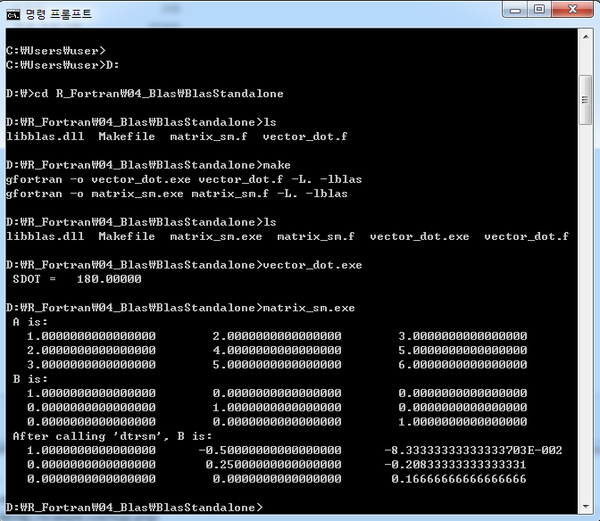## LAPACK (Linear Algebra PACKage)

LAPACK is written in Fortran 90 and provides routines for solving systems of simultaneous linear equations, least-squares solutions of linear systems of equations, eigenvalue problems, and singular value problems. The associated matrix factorizations (LU, Cholesky, QR, SVD, Schur, generalized Schur) are also provided, as are related computations such as reordering of the Schur factorizations and estimating condition numbers. Dense and banded matrices are handled, but not general sparse matrices. In all areas, similar functionality is provided for real and complex matrices, in both single and double precision.

Note: Check the Note information in BLAS section.

• Examples: Solving $$Ax=B$$
• Source location: gfortran/LapackStandalone/linear_equ.f
• Usage: Copy the downloaded libblas.dll and liblapack.dll to the R_Fortran/05_Lapack/LapackStandalone folder. In the CMD command line input make or directly input gfortran linear_equ.f -L. -llapack -o linear_equ.exe in the command line. The -L. -llapack option means link the liblapack.dll file in the current directory to the program. liblapack.dll depends on libblas.dll, so libblas.dll is also necessary.
• Reference: SGESV: http://www.netlib.org/lapack/single/sgesv.f
c     linear_equ.f
c     Example of solving linear equations
c     solving the matrix equation A*x=b using LAPACK
c     From: http://www.physics.orst.edu/~rubin/nacphy/lapack/codes/linear-f.html
Program LinearEquations
Implicit none
c     declarations, notice single precision
Real*4 A(3,3), b(3)
integer i, j, pivot(3), ok
c     define matrix A
data A/3.1, 1.0, 3.4, 1.3, -6.9, 7.2, -5.7, 5.8, -8.8/
c     define vector b, make b a matrix and you can solve multiple
c     equations with the same A but different b
data b/-1.3, -0.1, 1.8/
c     find the solution using the LAPACK routine SGESV
call SGESV(3, 1, A, 3, pivot, b, 3, ok)
c     parameters in the order as they appear in the function call
c     order of matrix A, number of right hand sides (b), matrix A,
c     leading dimension of A, array that records pivoting,
c     result vector b on entry, x on exit, leading dimension of b
c     return value
c
c     print the vector x
do i=1, 3
write(*,*) b(i)
end do
end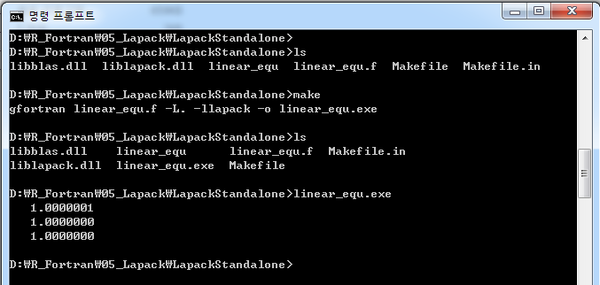## ATLAS (Automatically Tuned Linear Algebra Software)

ATLAS stands for Automatically Tuned Linear Algebra Software. ATLAS is both a research project and a software package. ATLAS's purpose is to provide portably optimal linear algebra software. The current version provides a complete BLAS API (for both C and Fortran77), and a very small subset of the LAPACK API. For all supported operations, ATLAS achieves performance on par with machine-specific tuned libraries.

# R foreign language interfaces

When to use? When a task requires explicit loops that can't be vectorized away. If a task can be vectorized or has no more than one loop, using R only would be a better choice, since in such situation, converting R code to C or Fortran doesn't improve much implementation efficient.

Tools? In linux system, if R has been installed, the developing environment is already prepared. In MS Windows system, after installing R, you also need to install the Rtools.

## Rtools

The standard toolchain for building R packages under Microsoft Windows, or for building R itself. It contains the command line tools, MinGW compilers, etc.

• Install
• Add path\to\Rtools\bin;path\to\Rtools\gcc-x.x.x\bin; to the system PATH variable
• For more details, read the Rtools.txt and README.txt file in the main Rtools directory.

## R and Fortran Interface

### .Fortran interface

#### General procedure

1. Writting Fortran subroutines, say xxx(arg1, arg2), and saving it in the foo.f file.
2. Compling Fortran code to Dynamic Link Library or shared objects(.dll/.so) with R CMD SHLIB foo.f command.
3. In R, loading foo.dll/foo.so to R with dyn.load('foo.dll')
4. In R, calling subroutines defined in foo.f with .Fortran function: .Fortran('xxx', arg1, arg2)

#### Notice

• The Fortran code to be called MUST be a subroutines.
• Lengths of the vectors passed to Fortran subroutine should be given.
• When invoking .Fortran function, argument types should be matched by explicit coersions with as.integer or as.double or by creating vectors of an explicit storage mode and length using the constructors integer(n) and double(n).
• The return value from .Fortran is a list with the same number of components (and names, if given) as the argument list.

Comparison of R and Fortran data types

R storage mode FORTRAN type
logical INTEGER
integer INTEGER
double DOUBLE PRECISION
complex DOUBLE COMPLEX
character CHARACTER*255
raw unsigned none

#### Example1: convolve

This is the canonical example introduced in Writting R Extensions.

The convolution of two sequences $$x_1, ..., x_n$$ and $$y_1, ...y_m$$ is defined as: $$z_k = \sum_{i+j=k}x_iy_j$$

The Fortran part

c A subroutine to convole two finite sequences
subroutine convolvef(a, na, b, nb, ab)
integer na, nb, nab, i, j
double precision a(na), b(nb), ab(na+nb-1)
nab = na + nb - 1

do 10 i = 1, nab
ab(i) = 0.0
10   continue
do 20 i = 1, na
do 30 j = 1, nb
ab(i+j-1) = ab(i+j) + a(i)*b(j)
30      continue
20   continue
end


The R part

# Load Shared Objects (*.so) / Dynamic-Link Library (*.DLL)
dyn.load(file.path("R_Fortran", "01_Convolve", paste0("convolve", .Platform$dynlib.ext))) # Platform independence # Check whether Fortran subroutine convolvef is available is.loaded("convolvef")  ##  TRUE  x=1:5 y=6:10 # .Fortran("convolvef", as.double(x), as.integer(length(x)), as.double(y), as.integer(length(y)), double(length(x) + length(y) - 1))  ## [] ##  1 2 3 4 5 ## ## [] ##  5 ## ## [] ##  6 7 8 9 10 ## ## [] ##  5 ## ## [] ##  6 19 40 70 110 114 106 85 50  .Fortran("convolvef", as.double(x), as.integer(length(x)), as.double(y), as.integer(length(y)), xy = double(length(x) + length(y) - 1))$xy

##    6  19  40  70 110 114 106  85  50

# R wrapper
conv_f <- function(x, y){
if(!(is.numeric(x) && is.numeric(y)))
stop("x and y should be both numeric vector")
.Fortran("convolvef", as.double(x), as.integer(length(x)), as.double(y), as.integer(length(y)),
double(length(x) + length(y) - 1))
}

conv_f(1:5, 6:10)

## []
##  1 2 3 4 5
##
## []
##  5
##
## []
##   6  7  8  9 10
##
## []
##  5
##
## []
##    6  19  40  70 110 114 106  85  50

conv_f(1:5, 6:7)

## []
##  1 2 3 4 5
##
## []
##  5
##
## []
##  6 7
##
## []
##  2
##
## []
##   6 19 32 45 58 35

dyn.unload(file.path("R_Fortran", "01_Convolve", paste0("convolve", .Platform$dynlib.ext))) # Platform independence  #### Example2: swap & Reverse We'll study more details about the .Fortran interface though this example. The Fortran part c swap two integer number subroutine ISWAPF (a, b) integer a, b, tmp tmp = a a = b b = tmp end c swap two double number subroutine DSWAPF (a, b) double precision a, b, tmp tmp = a a = b b = tmp end c Subroutine Reverse: c reverses the order of the input array subroutine Reversef(a, n) integer n, i double precision a(n) do 10 i = 1, INT(n/2) call DSWAPF(a(i), a(n - i + 1)) 10 continue end  The R part # ?.Fortran # All Fortran compilers known to be usable to compile R map # symbol names to lower case. Symbol names containing underscores are # not valid Fortran 77 (although they are valid in Fortran # 9x). Portable code should not use Fortran names containing underscores. # gfortran transforms names of entities specified in the Fortran source file by appending underscores to them. dyn.load(file.path("R_Fortran", "02_Swap", paste0("swap", .Platform$dynlib.ext)))

##  TRUE

x=10
y=20
# a and b are a copy of x and y, x and y doesn't change
# a and b are called by reference
.Fortran("iswapf", a=as.integer(x), b=as.integer(y))

## $a ##  20 ## ##$b
##  10

x; y

##  20

# The arguments are not meaningful
.Fortran("iswapf", p=as.integer(x), q=as.integer(y))

## $p ##  20 ## ##$q
##  10

# With 'DUP = FALSE', your compiled code can alter the local variable
# DEP=FALSE can speed up the program by avoiding pssing hudge matrix
# Oops..., didn't swap! Why?
.Fortran("iswapf", as.integer(x), as.integer(y), DUP=FALSE)

## []
##  20
##
## []
##  10

x; y

##  20

# Use dswapf. Wow!
.Fortran("dswapf", as.double(x), as.double(y), DUP=FALSE)

## []
##  20
##
## []
##  10

x; y

##  10

# Mhm...
is.double(x)

##  TRUE

x=as.integer(10)
y=as.integer(20)
.Fortran("iswapf", x, y, DUP=FALSE)

## []
##  20
##
## []
##  10

x; y

##  10

# Reverse a vector
# x, y are vectors of lenth n, exchange x[i] and y[i] if x[i] is bigger than y[i], i=1,...,n
# ivswapf subroutine invokes the iswapf subroutine
# Again, what's the problem here?
x=1:10
.Fortran("reversef", as.double(x), as.integer(length(x)), DUP=FALSE)

## []
##   10  9  8  7  6  5  4  3  2  1
##
## []
##  10

# So, always be careful about the dat type
is.integer(x)

##  TRUE

x=as.double(1:10)
.Fortran("reversef", x, as.integer(length(x)), DUP=FALSE)

## []
##   10  9  8  7  6  5  4  3  2  1
##
## []
##  10

x

##   10  9  8  7  6  5  4  3  2  1

dyn.unload(file.path("R_Fortran", "02_Swap", paste0("swap", .Platform$dynlib.ext)))  Concepts & Conclusions • symbol name vs transformed symbol name • the arguments are a copy of the passed variables • the arguments are 'meaningless' • passing the R variables' real address via DUP=FALSE • do coerce the data type before passing to Fortran #### Example3: array An example of using Fortran90 to calculate the summation and multiplication of matrix. Intrinsic functions in Fortran 90: http://www.nsc.liu.se/~boein/f77to90/a5.html The Fortran part ! subroutine of matrix summation with fortran90 subroutine arraysumf90(a, b, c, nrow, ncol) implicit none integer nrow, ncol, i, j double precision a(nrow, ncol), b(nrow, ncol), c(nrow, ncol) c = a + b end subroutine arraysumf90 ! subroutine of matrix inner product with fortran90 subroutine arraymulf90(a, b, c, nrow, ncol) implicit none integer nrow, ncol, i, j double precision a(nrow, ncol), b(nrow, ncol), c(nrow, ncol) c = matmul(a, b) end subroutine arraymulf90  The R part # Fortran90 x=y=z=matrix(1:9, 3) dyn.load(file.path("R_Fortran", "03_Array", paste0("array90", .Platform$dynlib.ext)))
# matrix summation

##  TRUE

.Fortran("arraysumf90", as.double(x), as.double(y), as.double(z), as.integer(nrow(x)), as.integer(ncol(x)))[]

##   2  4  6  8 10 12 14 16 18

# matrix multiplication

##  TRUE

.Fortran("arraymulf90", as.double(x), as.double(y), as.double(z), as.integer(nrow(x)), as.integer(ncol(x)))[]

##   30  36  42  66  81  96 102 126 150

x%*%y

##      [,1] [,2] [,3]
## [1,]   30   66  102
## [2,]   36   81  126
## [3,]   42   96  150

dyn.unload(file.path("R_Fortran", "03_Array", paste0("array90", .Platform$dynlib.ext)))  #### Example4: BLAS Working principle R uses the BLAS and LAPACK libraries to do basic linear algebra calculations, and the BLAS and LAPACK is used via Dynamic Link Library. On Windows, these DLL files, named Rblas.dll and Rlapack.dll, maybe exist in the bin folder under R home directory, such as R-x.xx/bin/x64. Fortran can use R's BLAS and Lapack library directly by linking to the DLLs, so you don't need to install BLAS and Lapack seperately on your system. To link your Fortran code to the DLLs, you need to use the -lblas and -llapack option whlie compiling your code to DLLs. Usually it would be convenient to write a Makevars file with the content: PKG_LIBS =$(LAPACK_LIBS) $(BLAS_LIBS)  Then when you run the R CMD SHLIB command, R can link your code to BLAS and Lapack properly. In this example, we'll make a fortran subroutine called idamaxf by using the IDAMAX function in BLAS library to calculate the first occurrence of the maximum absolute value of a double precision vector. Then compile it to DLL and invoke it in R. The Fortran part c IDAMAX searches a double precision vector for the first occurrence c of the the maximum absolute value. c Arguments: c n: Number of elements to process in the vector to be searched. c x: Array of dimension (n-1) * |incx| + 1. c incx: Increment between elements of x. subroutine idamaxf(n, x, incx, y) integer n, incx, y, idamax double precision x(*) y = idamax(n, x, incx) return end  The R part dyn.load(file.path("R_Fortran", "04_Blas", paste0("blas_idamax", .Platform$dynlib.ext)))
# Test

##  TRUE

n = 10
# PROBLEM: when incx < 0, return value is always 0!
incx = 1
x = sample((n-1)*abs(incx) + 1)
x

##    4  7 10  1  8  5  3  6  2  9

.Fortran("idamaxf", as.integer(n), as.double(x), as.integer(incx), as.integer(1))[]

##  3

# R wrapper
idamax <- function(x, n, by=1){
if(length(x) < ((n-1)*abs(by) +1))
stop("x is too short!")
.Fortran("idamaxf", as.integer(n), as.double(x), as.integer(by), as.integer(1))[]
}
(x=sample(10))

##    1  4  2  5  9  3 10  8  6  7

# which(x[1:5]==max(x[1:5]))
idamax(x, 5)

##  5

# which(x[seq(1,10,2)]==max(x[seq(1,10,2)]))
idamax(x, 5, by=2)

##  4

dyn.unload(file.path("R_Fortran", "04_Blas", paste0("blas_idamax", .Platform$dynlib.ext)))  #### Example5: LAPACK Working principle is the same with BLAS introduced in previous section. In this example, we'll make a subroutine called Lineareq to solve the equation $$Ax=b$$, where $$A$$ is a $$nxn$$ matrix and $$b$$ is a vector of length $$n$$, by using the DGESV subroutine in LAPACK library. The Fortran part c Example of solving linear equations c From: http://www.physics.orst.edu/~rubin/nacphy/lapack/codes/linear-f.html c SUBROUTINE: DGESV( N, NRHS, A, LDA, IPIV, B, LDB, INFO ) c http://www.netlib.org/lapack/single/sgesv.f c Solving the matrix equation A*x=b using LAPACK Subroutine Lineareq(A, b, n, info) Implicit none integer n, info integer ipiv(n) double precision A(n, n), b(n) call DGESV(n, 1, A, n, ipiv, b, n, info) end  The R part # Solving the matrix equation A*x=b using LAPACK dyn.load(file.path("R_Fortran", "05_Lapack", paste0("linear_equ", .Platform$dynlib.ext)))

##  TRUE

A = matrix(c(3.1, 1.3, -5.7, 1.0, -6.9, 5.8, 3.4, 7.2, -8.8), 3, byrow=TRUE)
b = c(-1.3, -0.1, 1.8)
solve(A)%*%b

##      [,1]
## [1,]    1
## [2,]    1
## [3,]    1

.Fortran("lineareq", A=as.double(A), b=as.double(b), n=as.integer(nrow(A)), info=as.integer(1))

## $A ##  3.4000 0.2941 0.9118 7.2000 -9.0176 0.5838 -8.8000 8.3882 -2.5737 ## ##$b
##  1 1 1
##
## $n ##  3 ## ##$info
##  0

# R wrapper
lineareq <- function(A, b){
if(!is.matrix(A))
stop("A must be a matrix")
if(nrow(A) != ncol(A))
stop("A must be a symmetic matrix")
if(nrow(A) != length(b))
stop("The row number of matrix 'A' is different with the length of vector 'b'")
res = .Fortran("lineareq",
A=as.double(A), b=as.double(b), n=as.integer(nrow(A)), info=as.integer(1))
if(res$info < 0) stop("Arguments error!") else if(res$info > 0)
stop("Singular!")
else
res$b } lineareq(A, b)  ##  1 1 1  dyn.unload(file.path("R_Fortran", "05_Lapack", paste0("linear_equ", .Platform$dynlib.ext)))


#### Example6: R API

There are a large number of entry points in the R executable/DLL that can be called from C code (and some that can be called from FORTRAN code)

The Fortran part

normal distribution pdf (version 1)

c    dnorm defined in R: dnorm(x, mean = 0, sd = 1, log = FALSE)
subroutine dnormf(x, mean, sd, log, re)
double precision x, mean, sd, re
integer log
call dnormwrap(x, mean, sd, log, re)
return
end


The corresponding C wrapper

#include <R.h>
#include <Rmath.h>
// DO NOT USE dnorm as the wrap function name.
void F77_SUB(dnormwrap)(double *x, double *mean, double *sd, int *log, double *re)
{
*re = Rf_dnorm4(*x, *mean, *sd, *log);
}


normal distribution pdf (version 2)

c    dnorm defined in R: dnorm(x, mean = 0, sd = 1, log = FALSE)
subroutine dnormf1(x, mean, sd, log, re)
double precision x, mean, sd, re, dnormwrap
integer log
re = dnormwrap(x, mean, sd, log)
return
end


The corresponding C wrapper

#include <R.h>
#include <Rmath.h>
// DO NOT USE dnorm as the wrap function name, since it is a kind of key words
double F77_SUB(dnormwrap)(double *x, double *mean, double *sd, int *log)
{
return Rf_dnorm4(*x, *mean, *sd, *log);
}


The R part

# fortran using R's dnorm function
# C wrapper version 1: void type, no return value
dyn.load(file.path("R_Fortran", "06_Rapi", "dnorm", paste0("dnorm", .Platform$dynlib.ext))) is.loaded("dnormf")  ##  TRUE  .Fortran("dnormf", as.double(0), as.double(0), as.double(1), as.integer(0), as.double(0))[]  ##  0.3989  dyn.unload(file.path("R_Fortran", "06_Rapi", "dnorm", paste0("dnorm", .Platform$dynlib.ext)))

# C wrapper version 2: double type, has return value
dyn.load(file.path("R_Fortran", "06_Rapi", "dnorm", paste0("dnorm1", .Platform$dynlib.ext))) is.loaded("dnormf1")  ##  TRUE  .Fortran("dnormf1", as.double(0), as.double(0), as.double(1), as.integer(0), as.double(0))[]  ##  0.3989  dyn.unload(file.path("R_Fortran", "06_Rapi", "dnorm", paste0("dnorm1", .Platform$dynlib.ext)))


The Fortran part

random number generation

c A subroutine generating normal & uniform random numbers
subroutine nurnd(x, y)
real*8 normrnd, unifrnd, x, y

call rndstart()
x = normrnd()
y = unifrnd()
call rndend()

return
end


The corresponding C wrapper

#include <R.h>
#include <Rmath.h>

void F77_SUB(rndstart)(void) { GetRNGstate(); }
void F77_SUB(rndend)(void) { PutRNGstate(); }
double F77_SUB(normrnd)(void) { return rnorm(0, 1); }
double F77_SUB(unifrnd)(void) { return runif(0, 1); }


The R part

# fortran using R's random number generation
dyn.load(file.path("R_Fortran", "06_Rapi", "random_number", paste0("random", .Platform$dynlib.ext))) is.loaded("nurnd")  ##  TRUE  .Fortran("nurnd", as.double(1), as.double(1))  ## [] ##  2.059 ## ## [] ##  0.286  dyn.unload(file.path("R_Fortran", "06_Rapi", "random_number", paste0("random", .Platform$dynlib.ext)))


## R and C Interface

Why?

• R itself is mainly written in C, consequently, C extensions fit perfectly with R.
• C is standardized, portable, and has good-quality free compilers on effectively all platforms.

There are two interfaces for C, .C and .Call

### .C interface

#### General procedure

1. Writting void type C functions, say xxx(arg1, arg2), and saving it in the foo.c file.
2. Compling C code to Dynamic Link Library or shared objects(.dll/.so) with R CMD SHLIB foo.c command.
3. In R, loading foo.dll/foo.so to R with dyn.load('foo.dll')
4. In R, calling subroutines defined in foo.c with .C function: .C('xxx', arg1, arg2)

#### Notice

• The C function should be void type and all values should be returned via arguments.
• All arguments should be passed as pointers, since all R data types are vectors.
• Lengths of the vectors passed to C function should be given.
• When invoking .C function, argument types should be matched by explicit coersions with as.integer or as.double or by creating vectors of an explicit storage mode and length using the constructors integer(n) and double(n).
• The return value from .C is a list with the same number of components (and names, if given) as the argument list.

Comparison of R and C data types

R storage mode C type
logical int *
integer int *
double double *
complex Rcomplex *
character char **
raw unsigned char *

#### Example1: convolve

The C part

void convolveC(double *a, int *na, double *b, int *nb, double *ab)
{
int i, j, nab = *na + *nb - 1;

for(i = 0; i < nab; i++)
ab[i] = 0.0;
for( i = 0; i < *na; i++)
for(j = 0; j < *nb; j++)
ab[i + j] += a[i]*b[j];
}


#### The R part

dyn.load(file.path("R_C", "01_convolve", paste0("convolveC", .Platform$dynlib.ext))) is.loaded("convolveC")  ##  TRUE  x=1:5 y=6:10 .C("convolveC", as.double(x), as.integer(length(x)), as.double(y), as.integer(length(y)), double(length(x) + length(y) -1))  ## [] ##  1 2 3 4 5 ## ## [] ##  5 ## ## [] ##  6 7 8 9 10 ## ## [] ##  5 ## ## [] ##  6 19 40 70 110 114 106 85 50  # R wrapper conv_C <- function(x, y){ if(!(is.numeric(x) && is.numeric(y))) stop("x and y should be both numeric vector") .C("convolveC", as.double(x), as.integer(length(x)), as.double(y), as.integer(length(y)), double(length(x) + length(y) - 1))[] } conv_C(1:5, 6:10)  ##  6 19 40 70 110 114 106 85 50  conv_C(1:5, 6:7)  ##  6 19 32 45 58 35  dyn.unload(file.path("R_C", "01_convolve", paste0("convolveC", .Platform$dynlib.ext)))


### .Call

Features

• Passing R objects to C
• Creating R objects in C
• Manipulating R objects in C
• Returning R objects from C

#### syntax

SEXP FunctionName(SEXP arg1, SEXP arg2, ...)

• SEXP standing for S expression, is a pointer to a C struct.
• The returned value from C is also a SEXP type.
• If new SEXP objects are created within the C function, they should be protected with PROTECT to keep them from being cleaned by R as garbage.
• Header file "Rinternals.h" should be included.

#### Example1: convolve

The C part

#include <Rinternals.h>

SEXP convolveCall(SEXP a, SEXP b)
{
int na, nb, nab;
double *xa, *xb, *xab;
SEXP ab;

a = PROTECT(coerceVector(a, REALSXP));
b = PROTECT(coerceVector(b, REALSXP));
na = length(a); nb = length(b); nab = na + nb - 1;
ab = PROTECT(allocVector(REALSXP, nab));
xa = REAL(a); xb = REAL(b); xab = REAL(ab);
for(int i = 0; i < nab; i++) xab[i] = 0.0;
for(int i = 0; i < na; i++)
for(int j = 0; j < nb; j++) xab[i + j] += xa[i] * xb[j];
UNPROTECT(3);
return ab;
}


#### The R part

dyn.load(file.path("R_C", "01_convolve", paste0("convolveCall", .Platform$dynlib.ext))) is.loaded("convolveCall")  ##  TRUE  x=1:5 y=6:10 .Call("convolveCall", x, y)  ##  6 19 40 70 110 114 106 85 50  dyn.unload(file.path("R_C", "01_convolve", paste0("convolveCall", .Platform$dynlib.ext)))


## Speed comparison

We use the convolve example to compare the speed of all the R interfaces.

## Compare the speed of C and Fortran interfaces with R
dyn.load(file.path("R_Fortran", "01_convolve", paste0("convolve", .Platform$dynlib.ext))) # Platform independence dyn.load(file.path("R_C", "01_convolve", paste0("convolveC", .Platform$dynlib.ext)))
dyn.load(file.path("R_C", "01_convolve", paste0("convolveCall", .Platform$dynlib.ext))) convolveR <- function(x, y){ nx <- length(x) ny <- length(y) z <- double(nx + ny -1) for(i in 1:nx) for (j in 1:ny) z[i + j - 1] = z[i + j-1] + x[i]*y[j] z } convolveF <- function(x, nx, y, ny){ .Fortran("convolvef", as.double(x), as.integer(nx), as.double(y), as.integer(ny), double(nx + ny - 1)) } convolveC <- function(x, nx, y, ny){ .C("convolveC", as.double(x), as.integer(nx), as.double(y), as.integer(ny), double(nx + ny - 1)) } convolveCall <- function(x, y){ .Call("convolveCall", x, y) } library(rbenchmark) set.seed(1204) x = rnorm(1000); y = rnorm(1000) nx = length(x); ny = length(y) benchmark(convolveF = convolveF(x, nx, y, ny), convolveC = convolveC(x, nx, y ,ny), convolveCall = convolveCall(x, y), replications=1000, columns=c('test', 'replications', 'elapsed', 'relative'))  ## test replications elapsed relative ## 2 convolveC 1000 1.02 1.500 ## 3 convolveCall 1000 0.98 1.441 ## 1 convolveF 1000 0.68 1.000  system.time(convolveR(x, y))  ## user system elapsed ## 4.2 0.0 4.2  dyn.unload(file.path("R_Fortran", "01_convolve", paste0("convolve", .Platform$dynlib.ext)))  # Platform independence
dyn.unload(file.path("R_C", "01_convolve", paste0("convolveC", .Platform$dynlib.ext))) dyn.unload(file.path("R_C", "01_convolve", paste0("convolveCall", .Platform$dynlib.ext)))


## R and C++ Interface

### Rcpp

Selected Sources:

Fibonacci example

## basic R function
fibR <- function(n) {
if (n == 0) return(0)
if (n == 1) return(1)
return (fibR(n - 1) + fibR(n - 2))
}

library(Rcpp)
library(inline)
## we need a pure C/C++ function here
incltxt <- '
int fibonacci(const int x) {
if (x == 0) return(0);
if (x == 1) return(1);
return (fibonacci(x - 1)) + fibonacci(x - 2);
}'
## Rcpp version of Fibonacci
fibRcpp <- cxxfunction(signature(xs="int"),
plugin="Rcpp",
incl=incltxt, body='
int x = Rcpp::as(xs);
return Rcpp::wrap( fibonacci(x) );
')

## cygwin warning:
##   MS-DOS style path detected: D:/PROGRA~1/R-30~1.2/etc/x64/Makeconf
##   Preferred POSIX equivalent is: /cygdrive/d/PROGRA~1/R-30~1.2/etc/x64/Makeconf
##   CYGWIN environment variable option "nodosfilewarning" turns off this warning.
##   Consult the user's guide for more details about POSIX paths:
##     http://cygwin.com/cygwin-ug-net/using.html#using-pathnames

library(rbenchmark)
N = 20
res <- benchmark(fibR(N), fibRcpp(N),
columns=c("test", "replications", "elapsed",
"relative", "user.self", "sys.self"),
order="relative", replications=100)
res

##         test replications elapsed relative user.self sys.self
## 1    fibR(N)          100    5.96       NA      5.96        0
## 2 fibRcpp(N)          100    0.00       NA      0.00        0Armadillo is a C++ linear algebra library (matrix maths) aiming towards a good balance between speed and ease of use.

# Building R packages

## Tools for Windows

### Rtools

See the R foreign language interfaces/Rtools section for details.

### MiKTex (optional)

A LaTeX and pdftex package used to build .pdf forms of documentation.

• The PATH is automatically set during the installation. Use pdflatex --version in the command line to test whether MiKTeX is installed successfully.

## R package structure

The simplest package structure is:

pkg (the package name)
|
|--DESCRIPTION (contains basic information about the package)
|--NAMESPACE (controls which variables to export, import, etc.)
|--R (R source files)
|--function1.R
|--function2.R
|--...
|--man (help documents)
|--function1.Rd
|--function2.Rd
|--...
|--...


Optional subdirectories are data, demo, exec, inst, po, src, tests, tools and vignettes.

## Example

In the traditional way of making package, you need to write R functions (say myfun) first and then add the correponding help files (myfun.Rd) to the man directory. However, it is an error-prone process. Here I'll introduce a more advanced process using Roxygen2 package.

As an example, we'll make a package called MyPkg here.

1. Make a folder called Mypkg
2. Make a file called DESCRIPTION, with the following content:

Package: MyPkg
Type: Package
Title: A package demo
Version: 1.0
Date: 2013-12-06
Author: Weicheng Zhu
Maintainer: Weicheng <weicheng@dreamhunter.me>
Description: More about what it does (maybe more than one line)

3. Make a folder named R under Mypkg

4. Make a file named myFun.R under Mypkg

5. Add the convolvR function to myFun.R:

##' A description of this function
##'
##' @title Convolution of two vector
##' @param x numeric vector
##' @param y numeric vector
##' @return numeric vector of length (nx+ny-1)
##' @author weicheng
##' @export
convolveR <- function(x, y){
nx <- length(x)
ny <- length(y)
z <- double(nx + ny -1)
for(i in 1:nx)
for (j in 1:ny)
z[i + j - 1] = z[i + j-1] + x[i]*y[j]
z
}


Now, your directory structure would be like this:

MyPkg
|--DESCRIPTION
|--R
|--myFun.R

6. Run R and set the working direcotry to the parent direcotry of MyPkg, then run the following command:

library(roxygen2)
roxygenize("MyPkg")


After running this commands, the directory structure of the MyPkg would be like this:

MyPkg
|--DESCRIPTION
|--man
|--convolveR.Rd
|--NAMESPACE
|--R
|--myFun.R

7. In the system command line, go the parent directory of MyPkg and run R CMD build MyPkg, then a file named MyPkg_1.0.tar.gz will be made. Then run R CMD check MyPkg_1.0.tar.gz, if no error occurs this R package is made successfully and can be installed with the command R CMD INSTALL MyPkg_1.0.tar.gz.

8. Test MyPkg in R:

library(MyPkg)
help(package="MyPkg")
convolveR(1:5,1:5)


## Building R package using Rcpp and RcppArmadillo

Rcpp and RcppArmadillo both provide a package skeleton function, which makes building R packages more easier.

library(Rcpp)
Rcpp.package.skeleton()


library(RcppArmadillo)